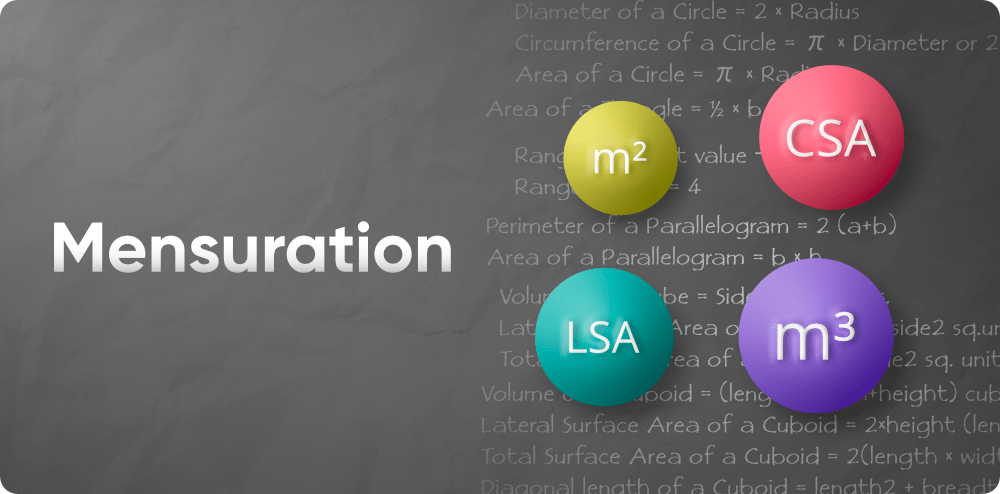# Mensuration

• Difficulty Level : Expert
• Last Updated : 30 May, 2022

Mensuration is a branch of mathematics concerned with the calculation of geometric figures and their parameters such as weight, volume, form, surface area, lateral surface area, and so on. In simple mathematics, you can learn about mensuration. The principles of mensuration are discussed here, as are all the essential mensuration formulas.For a better understanding of these ideas, the properties of various geometric forms and the accompanying figures are also shown. Mensuration is a branch of mathematics that deals with the scale, volume, or area of various geometric forms. These forms are available in two or three dimensions.

### Mensuration of 2D shapes

Mensuration is the measuring theory. It is the field of mathematics that is used to measure various figures such as the cube, cuboid, square, rectangle, cylinder, and so on. Mensuration of two-dimensional figures such as area, perimeter, and so on. The form or figure having two dimensions, such as length and breadth, is referred to as a 2-D form. A 2-D figure is something like a square, rectangle, triangle, parallelogram, trapezium, rhombus, and so on. We can measure 2-D forms using Area (A) and Perimeter (P) as discussed below:

### Mensuration of 3D shapes

Mensuration is concerned with the measuring of three-dimensional solids in terms of total surface area, lateral/curved surface area, and volume. 3D figures are those that have more than two dimensions, such as length, breadth, and height. Cube, Cuboid, Sphere, Cylinder, Cone, and other three-dimensional forms are examples. Total Surface Area, Lateral Surface Area, Curved Surface Area, and Volume are used to calculate the 3D figure are discussed in the articles below:

My Personal Notes arrow_drop_up
Recommended Articles
Page :